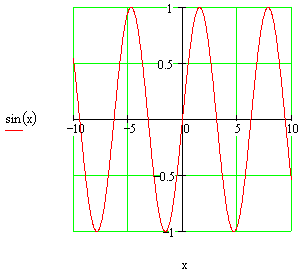# The Graph of the Sine FunctionTo the left is the graph of the sine function.

Click the EZ Graph link below to examine this graph with a graphic calculator, explanations included. Links for other examples of the sine function graph along with their explanations follow below.

More graphs of the sine function using EZ Graph:

Angle in degrees: